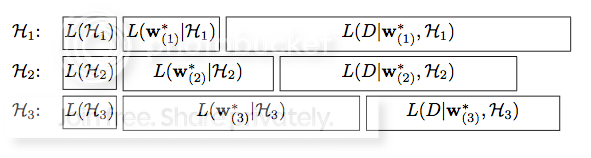### Motivation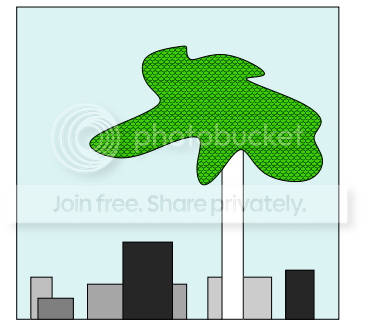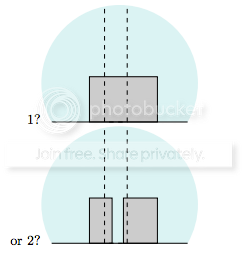$-x^{3}/11 + 9/11x^{2} + 23/11$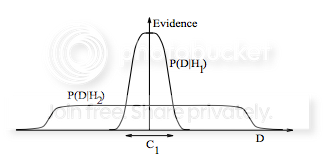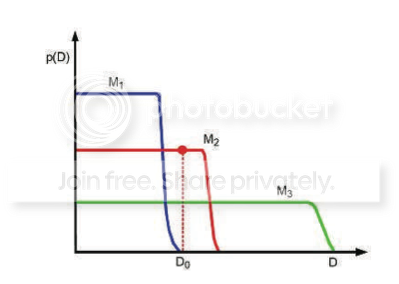A theory with mathematical beauty is more likely to be correct than an ugly one that fit some experimental data.

### Theoretical explanation

#### Bayesian Model Selection

$$p(\theta \mid D ,m_{i} ) = \frac{p(D \mid \theta, m_{i} )p(\theta \mid m_{i})}{ p(D \mid m_{i} )}$$

$$p(m_{i} \mid D) \propto p(D \mid m_{i}) \times p(m_{i})$$

$$p(D \mid m_{i}) \approx p(D \mid \theta_{MP}, m_{i}) \times p(\theta_{MP} \mid m_{i})\sigma_{\theta \mid D}$$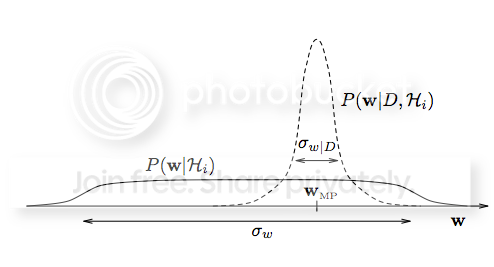$$Occam Factor = \frac{\sigma_{\theta \mid D}}{\sigma_{\theta}}$$

The Occam factor is equal to the ratio of the posterior accessible volume of $m_{i}$’s parameter space to the prior accessible volume.

#### Minimum description length(MDL)

$$P(x) = 2^{-L(x)}, L(x) = -log_{2}{P(x)}$$

$$L(D, M) = -logP(M) - logP(D \mid M)$$